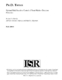## Optimal Risk Sensitive Control of Semi-Markov Decision Processes##### Files
PhD_2000-8.pdf(1.08 MB)
In this thesis, we study risk-sensitive cost minimization in semi-Markov decision processes. The main thrust of the thesis concerns the minimization of average risk sensitive costs over the infinite horizon. <p>Existing theory is expanded intwo directions: the semi-Markov case is considered, and non-irreduciblechains are considered. In particular, the analysis of the non-irreduciblecase is a significant addition to the literature, since many real-worldsystems do not exhibit irreducibility under all stationary Markov policies. Extension of existing results to the semi-Markovcase is significant because it requires the definition of a newdynamic programming equation and a technically challenging adaptation of the Perron-Frobeniuseigenvalue from the discrete time case.<p>In order to determine an optimal policy, new concepts in the classificationof Markov chains need to be introduced. This is because in thenon-irreducible case, the average risk sensitive cost objective function permits extremely unlikely events to exert a controlling influence on costs. We define equivalence classes of statescalled strongly communicating classes' and formulate in terms of thema new characterization of the underlying structureof Markov Decision Problems and Markov chains.<p>In the risk sensitive case, the expected cost incurred prior to a stopping time with finite expected valuecan be infinite. For this reason, we introduce an assumption: reachability with finite cost. This is the fundamental assumptionrequired to achieve the major results of this thesis.<p>We explore existence conditions for an optimal policy, optimality equations,and behavior for large and small risk sensitivity parameter. (Onlynon-negative risk parameters are discussed in this thesis -- i.e. the risk averse and risk neutral cases, not the risk seeking case.) Ramificationsfor the risk neutral objective function are also analyzed.Furthermore, a simple solution technique we call recursive computation'to find an optimal policy that isapplicable to small state spaces is described through examples.<p>The countable state space case is explored, and results that hold only for a finite state space are also presented. Other, relatedobjective functions such as sample path cost are analyzed and discussed.<p>We also explore finite time horizon semi-Markov problems, and present a general technique for solving them.We define a new objective function, the minimization of which is calledthe `deadline problem'. This is a problem in which the probability of reaching the goal state in a set period of time is maximized. We transform thedeadline problem objective function into an equivalent finite-horizonrisk sensitive objective function.